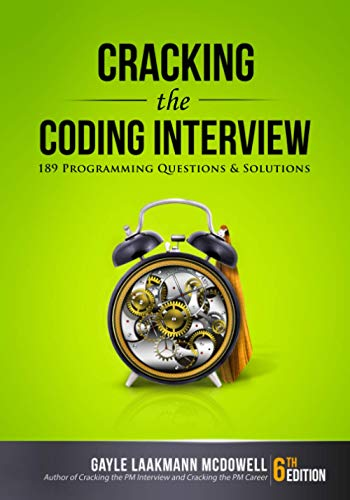# Chasing DogsQuestion: There are four dogs each at the corner of a unit square. Each of the dogs starts chasing the dog in the clockwise direction. They all run at the same speed and continuously change their direction accordingly so that they are always heading straight towards the other dog. How long does it take for the dogs to catch each other and where?

Answer: Let the dogs be A, B, C and D where A is chasing B, B is chasing C, C is chasing D and D is chasing A.

All the dogs will eventually meet in the center of the square. Since all the dogs move in symmetry, the only logical answer to the location of their meeting is the center of the square.

At any point in time, dog A is perpendicular to dog B and B perpendicular to C and so on. Dog A moves towards dog B but dog B does not move towards or away from dog A since it is moving perpendicular to dog A. Therefore, the distance that dog A needs to cover to reach dog B is the same as the original distance between them, one unit.

The speed of each of the dog towards the dog it is chasing is given by (1 + cos(t)) where t is the angle on each corner of the square.

Speed of dog = 1 + cos(90) = 1 + 0 = 1
Time needed = Distance/Speed = 1 / 1 = 1 unit.

Liked this question? Post this on Stumble, Twitter or Digg!

Have a better solution? Let us know through the comments section!

If you're looking for some serious preparation for your interviews, I'd recommend this book written by a lead Google interviewer. It has 189 programming questions and solutions:## 5 Responses

1.Alpha Simon says:

consider the problem of 3 dogs and a equilateral triangle then i think 1+cos(t) will fail.
As according to me time will be 1 unit only.

2.Bamadeva says:

If each dog turns at the mid line they need to go half unit and they will meet at the center where two diagonals of the square meets.

Let me know whether it is correct or not please.

3.Mathematician says:

That they meet at the center of the square can be shown more rigorously as follows:
Let Ax(t) and Ay(t) be functions of time which provide the x and y coordinates of dog A at time t. Assume the square is centered at the origin. Since dog B is at a 90 degree rotation of dog A and will keep that position all the time due to symmetry: Bx(t)=Ay(t) and By(t)=-Ax(t). When dog A reaches dog B, by definition we must have:
Ax(t) = Bx(t) and Ay(t)=By(t)
From the above we conclude that at the time dog A reaches dog B:
Ax(t) = Ay(t) = 0
which means that they reach each other at the origin which is the center of the square.

4.Mathematician says:

The first answer, that they meet in the center of the square, is correct though arrived at informally. The second answer is laughably wrong. Obviously the time is a function of speed. The faster the dogs, the sooner they will catch one another.

5.JJ says:

Still I didn’t understand the problem!
How could you able to write a statement like : “The speed of each of the dog towards the dog it is chasing is given by (1 + cos(t))…” ?

Can you just elaborate the solution ?

XHTML: These are some of the tags you can use: `<a href=""> <b> <blockquote> <code> <em> <i> <strike> <strong>`